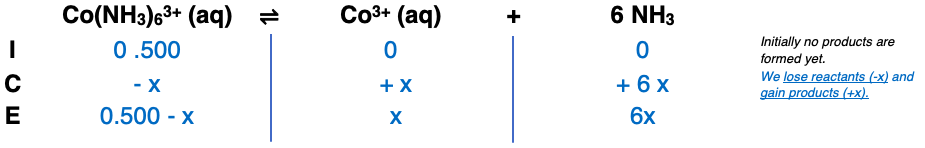# Problem: Using the dissociation constant, Kd = 2.2 × 10–34, calculate the equilibrium concentrations of Co3+ and NH3 in a 0.500-M solution of Co(NH3)63+.

###### FREE Expert Solution86% (456 ratings)###### Problem Details

Using the dissociation constant, Kd = 2.2 × 10–34, calculate the equilibrium concentrations of Co3+ and NH3 in a 0.500-M solution of Co(NH3)63+.